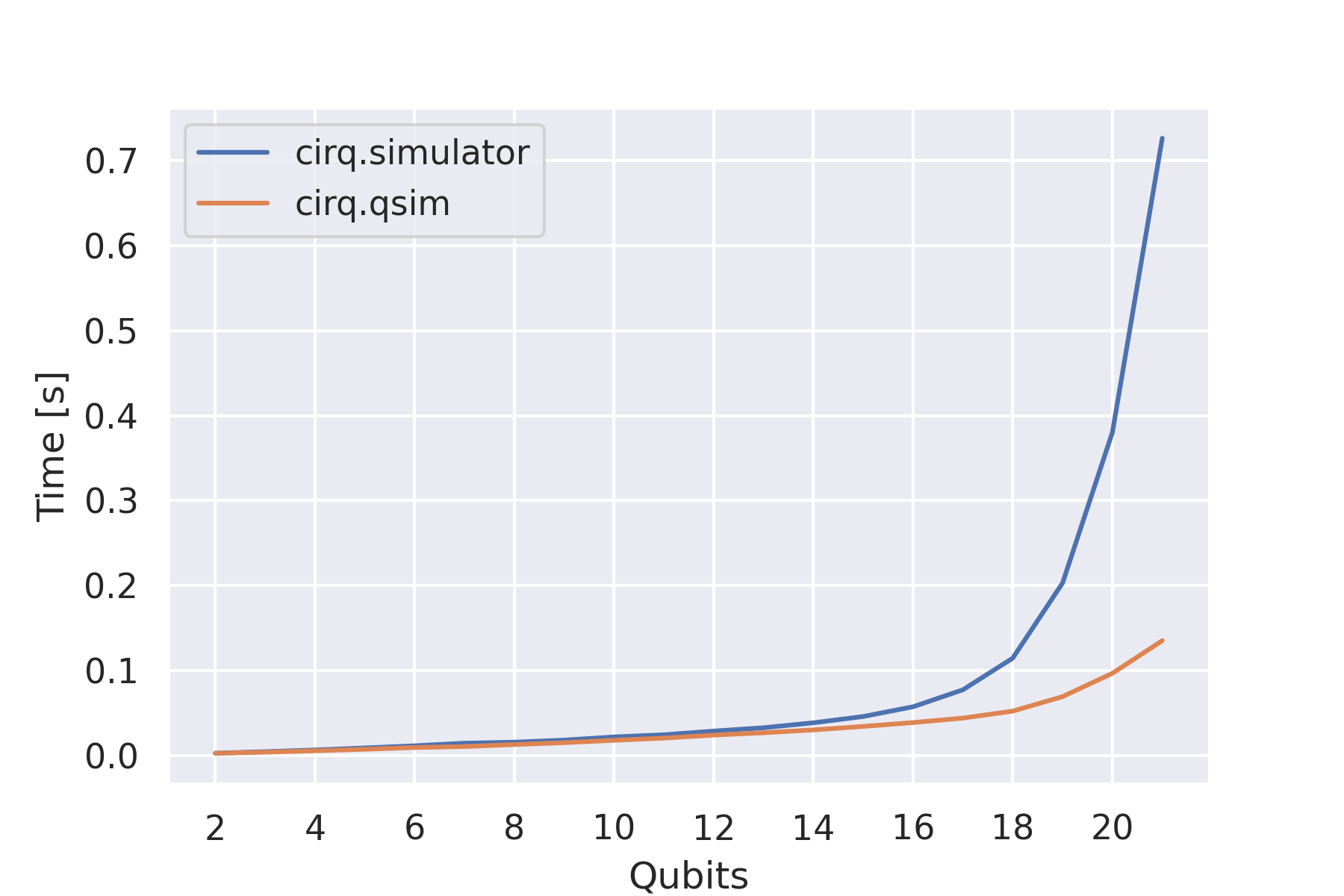# The qsim device¶

The qsim circuit simulator package provides two additional circuit simulators that can be used with PennyLane-Cirq:

• qsim, a Schrödinger full state-vector simulator

• qsimh, a hybrid Schrödinger-Feynman simulator. This simulator cuts the qubit lattice into two parts; each part is individually simulated using qsim, with Feynman-style path summation used to return the final result. Compared to full state-vector simulation, qsimh reduces memory requirements, at the expense of an increased runtime.

For further details see the qsim website.

For a large number of qubits, these simulators are considerably faster than the Cirq simulator:In order to use these devices, the qsimcirq package must first be installed:

pip install qsimcirq


You can instantiate the qsim device in PennyLane as follows:

import pennylane as qml

dev = qml.device('cirq.qsim', wires=2)


This device can then be used just like other devices for the evaluation of QNodes within PennyLane. A simple quantum function that returns the expectation value of a measurement and depends on three classical input parameters would look like:

@qml.qnode(dev)
def circuit(x, y, z):
qml.RZ(z, wires=)
qml.RY(y, wires=)
qml.RX(x, wires=)
qml.CNOT(wires=[0, 1])
return qml.expval(qml.PauliZ(wires=1))


You can then execute the circuit like any other function to get the quantum mechanical expectation value:

circuit(0.2, 0.1, 0.3)


The qsimh device can be loaded similarly, with the addition of the keyword argument qsimh_options as described below.

## Device options¶

qsim and qsimh use the same method of defining qubits as Cirq, e.g., LineQubit or GridQubit. As with the Cirq device, the qsim and qsimh devices therefore accept an additional argument qubits that can be used to define your own qubits and pass them to the device as a list.

import cirq

qubits = [
cirq.GridQubit(0, 0),
cirq.GridQubit(0, 1),
cirq.GridQubit(1, 0),
cirq.GridQubit(1, 1),
]

dev = qml.device("cirq.qsim", wires=4, qubits=qubits)


The wire of each qubit corresponds to its index in the qubit list. In the above example, the wire 2 corresponds to cirq.GridQubit(1, 0).

If no qubits are given, the plugin will create an array of LineQubit instances.

### qsimh options¶

qsimh requires specific options to be set for the simulator. These can be passed by the positional argument qsimh_options. See the qsimh usage documentation for further details.

import cirq

qubits = [
cirq.GridQubit(0, 0),
cirq.GridQubit(0, 1),
cirq.GridQubit(1, 0),
cirq.GridQubit(1, 1),
]

qsimh_options = {
'k': ,
'w': 0,
'p': 0,
'r': 2
}

dev = qml.device("cirq.qsimh", wires=4, qsimh_options=qsimh_options, qubits=qubits)


## Supported operations¶

The cirq.qsim and cirq.qsimh devices support most PennyLane operations and observables, with the exceptions of most adjoint operations and QubitUnitary gates on 3 or more qubits.

For state preparation qsim relies on decomposing BasisState into a set of PauliX gates and QubitStateVector via Möttönen state preparation.